## Posts Tagged ‘cho u’

### Kisei 2011- Cho U Equals the Score in Game 2

Saturday, January 29th, 2011

After losing the first game to challenger Iyama Yuta, Kisei title holder Cho U won the second game.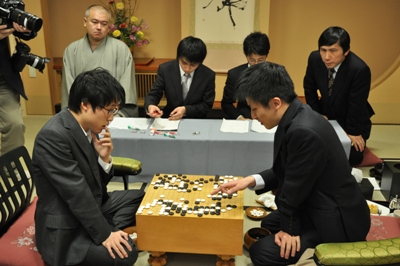I found Iyama’s move 40 quite amazing, it is played in a place that would never occur to me, see the next diagram.In the game black replied by connecting with the triangle marked stone. This makes move 40 a very efficient forcing move, since now white has even more forcing moves from the outside. This diagram shows the strongest continuation for white – in fact white did play this, but much later in the game.The last diagram shows why black cannot reply aggressively in response to white 40.Here is the complete game.

(; EV[35th Kisei title match 2] KM[6.5] FF SZ GM PW[Iyama Yuta] WR[Meijin] DT[2011-01-26,27] BR[Kisei] RE[B+R] PB[Cho U] ;B[qd];W[dc];B[pq];W[cp];B[nc];W[po];B[qm];W[qq];B[mq];W[pp];B[pr] ;W[ro];B[eq];W[do];B[pj];W[qr];B[ip];W[ci];B[fd];W[hd];B[dd];W[ed] ;B[ee];W[ec];B[cc];W[fe];B[cd];W[ff];B[ef];W[fc];B[cg];W[eg];B[dg] ;W[eh];B[eo];W[en];B[fo];W[dq];B[er];W[be];B[df];W[jp];B[jo];W[kp] ;B[mo];W[io];B[ho];W[in];B[iq];W[kn];B[hn];W[im];B[dn];W[cn];B[dm] ;W[cm];B[dl];W[cl];B[ph];W[ld];B[me];W[qc];B[pc];W[le];B[mf];W[lf] ;B[lg];W[md];B[mh];W[nd];B[od];W[rd];B[rc];W[ob];B[qb];W[ng];B[nh] ;W[kg];B[qn];W[mn];B[qo];W[qp];B[no];W[nn];B[lp];W[ko];B[kr];W[mg] ;B[lh];W[og];B[pg];W[oe];B[oc];W[pf];B[qf];W[qe];B[re];W[pe];B[rg] ;W[kh];B[lb];W[bg];B[bh];W[ah];B[bf];W[af];B[ag];W[rf];B[sf];W[bg] ;B[bi];W[cf];B[ch];W[ce];B[bd];W[de];B[ad];W[ae];B[ai];W[jr];B[jq] ;W[cb];B[ag];W[kq];B[lr];W[ah];B[bb];W[ac];B[bc];W[ba];B[ab];W[oj] ;B[ok];W[db];B[ag];W[pk];B[pl];W[ah];B[dk];W[ca];B[ag];W[nk];B[qk] ;W[ah];B[bo];W[aa];B[co];W[bk];B[ck];W[bj];B[cj];W[di];B[bm];W[bl] ;B[bn];W[dh];B[ag];W[ac];B[bf];W[mb];B[al];W[mc];B[nb];W[na];B[rn] ;W[mj];B[oi];W[gl];B[fk];W[gk];B[on];W[oq];B[or];W[nr];B[nq];W[op] ;B[ns];W[ps];B[mr];W[sp];B[qs];W[rr];B[sn];W[sq];B[fi];W[gh];B[lj] ;W[lk];B[ki])

### Kisei 2011 – Iyama Yuta Wins Game 1

Friday, January 14th, 2011

Iyama Yuta who challenges Cho U for the Japanese Kisei title won game one by resignation.Here is the exchange that took place at the end of the game:The continuation is puzzling though – did Cho U make a mistake, or just set the scene for resignation? Here are the final moves. After black cuts with 6, a and b are miai for black, and he wins the semeai on the upper side.White should have played as in the following diagram. The game looks close to me after this – in fact white looks a little ahead, but maybe I am missing something.Here is the complete game.

(;FFGMSZSTCA[UTF-8]AP[SGFC:1.16] EV[Kisei 2011, game 1] PB[Iyama Yuta] BR[9DP] HA PW[Cho U] WR[9DP] KM[6.5] DT[2011-01-14] RE[B+R] ;B[pd];W[dp];B[qp];W[ed];B[cd];W[cc];B[ic];W[dd];B[ce];W[bc] ;B[cn];W[fp];B[dj];W[oq];B[kq];W[on];B[qm];W[iq];B[ko];W[ol] ;B[pk];W[ok];B[pj];W[oj];B[pi];W[jp];B[kp];W[km];B[jo];W[im] ;B[ho];W[gq];B[hr];W[ir];B[hm];W[in];B[io];W[hn];B[hq];W[gr] ;B[fo];W[hp];B[gn];W[dn];B[dm];W[en];B[il];W[jl];B[jm];W[jn] ;B[kn];W[jm];B[lm];W[ik];B[lk];W[mn];B[ln];W[hl];B[gm];W[fl] ;B[po];W[oo];B[pq];W[pr];B[or];W[nr];B[gp];W[go];B[op];W[nq] ;B[gp];W[hs];B[ep];W[go];B[np];W[mp];B[gp];W[fq];B[eo];W[do] ;B[eq];W[go];B[er];W[dl];B[cq];W[lr];B[kr];W[js];B[mq];W[no] ;B[pp];W[lq];B[lp];W[mo];B[gp];W[cm];B[ls];W[mr];B[go];W[ks] ;B[dg];W[dq];B[dr];W[bq];B[cp];W[co];B[bp];W[bo];B[br];W[gc] ;B[nc];W[ci];B[di];W[cj];B[ch];W[eh];B[ck];W[bk];B[eg];W[fh] ;B[fg];W[gh];B[gg];W[hg];B[hf];W[if];B[he];W[ig];B[ie];W[qd] ;B[qe];W[pc];B[od];W[rc];B[re];W[ob];B[nb];W[qb];B[qr];W[dh] ;B[bh];W[bj];B[hj];W[hi];B[gl];W[ij];B[gk];W[fj];B[rd];W[qc] ;B[hb];W[gb];B[jf];W[jg];B[kf];W[mg];B[kg];W[kh];B[ps];W[pl] ;B[ql];W[pn];B[qn];W[me];B[og];W[ng];B[lh];W[oh];B[ki];W[of] ;B[jh];W[ii];B[pg];W[oi];B[ph];W[ld];B[lc];W[hc];B[ib];W[kc] ;B[kb];W[jd];B[jc];W[kd];B[id];W[mc];B[fk];W[ek];B[lb];W[ae] ;B[fm];W[bf];B[em];W[dk];B[fd];W[fe];B[gd];W[ee];B[ge];W[cf] ;B[eb];W[fc];B[ec];W[db];B[ea];W[ga];B[dc])

### Kisei 2011 Started

Thursday, January 13th, 2011

The most important Japanese title in professional Go, Kisei, started yesterday.

The title holder is Cho U, while the challenger is Iyama Yuta who seems to be in very good shape recently (he defeated Cho U in the final of the Daiwa Cup).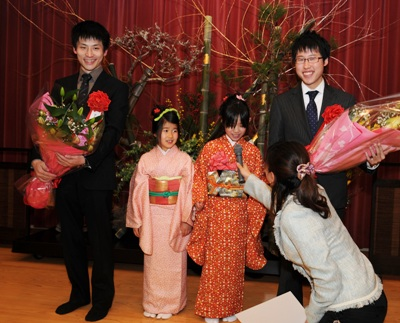Here is the game at the end of day one – Cho U plays white and he made the sealed move. White’s shape in the center looks bad, like damezumari, but he can capture some black stones and fix that.### Cho U – Yamashita Keigo 1-0 in Judan Title Match

Thursday, March 4th, 2010

Just a few days after Cho U captured number one Japanese title, Kisei, from Yamashita Keigo the same two met again in the first game of the Judan title. This time Cho U is the title holder and Yamashita Keigo is the challenger.

Cho U captured Judan from Takao Shinji last year. Takao then put up a good fight in the qualification tournament to become challenger, but was defeated by Yamashita in the final of the knock-out tournament that decided the challenger.

Cho U started his defense of the Judan title well and won the first game. The next game will be played on March 25th.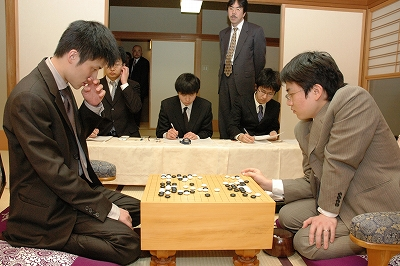Here is the game record: Cho U played black and won by resignation.

(; KM[6.5] EV[48th Judan title match 1] FF SZ GM PW[Yamashita Keigo] WR[Tengen] DT[2010-03-04] BR[Judan] RE[B+R] PB[Cho U] ;B[pd];W[dd];B[qp];W[dq];B[co];W[op];B[oq];W[nq];B[pq];W[dl];B[np] ;W[mq];B[no];W[kp];B[iq];W[fp];B[cq];W[dp];B[io];W[cp];B[lo];W[ip] ;B[jp];W[ko];B[hp];W[km];B[kq];W[lp];B[pj];W[nn];B[mo];W[ll];B[on] ;W[nc];B[lj];W[om];B[nl];W[mk];B[nm];W[mj];B[pf];W[mh];B[jd];W[hd] ;B[me];W[ce];B[jf];W[hf];B[cc];W[dc];B[hc];W[gc];B[ic];W[pb];B[qc] ;W[lc];B[gb];W[fc];B[nd];W[le];B[ld];W[kd];B[md];W[kc];B[ke];W[mc] ;B[lf];W[dj];B[cm];W[cl];B[il];W[jj];B[bp];W[bq];B[bl];W[bk];B[bn] ;W[kn];B[qb];W[ie];B[je];W[oc];B[dm];W[em];B[en];W[fm];B[fn];W[gm] ;B[br];W[cr];B[aq];W[go];B[ck];W[al];B[bm];W[dk];B[cj];W[bj];B[ci] ;W[bi];B[gn];W[hn];B[ho];W[hm];B[eo];W[gr];B[ep];W[hr];B[fq];W[fr] ;B[eq];W[er];B[ok];W[cq];B[ig];W[hh];B[ji];W[ii];B[hg];W[gg];B[ki] ;W[ih];B[jh];W[kk];B[cb];W[oi];B[pi];W[db];B[bd];W[ph];B[qh];W[be] ;B[ca];W[od];B[jb];W[kb];B[gf];W[gh];B[pc];W[fb];B[if])

### Cho U Captures Kisei Title!

Thursday, February 25th, 2010

Game five of the 34th Kisei title resulted in Cho U’s win, which ensured his capturing of the title from Yamashita Keigo by a 4-1 score. Yamashita captured the Kisei title in 2006 from Hane Naoki, then defended it for 3 consecutive years.

It looks like Cho U’s bad form from 2009 has not followed him in 2010.Here is the game record from the last game: Cho U played white and won by 1.5 points.

(;CA[Windows-1252]SZAP[MultiGo:4.4.4]GN[34th Kisei Title, game 5]EV[34th Kisei Title] DT[2010-02-26]PB[Yamashita Keigo]BR[9p]BT[Japan]PW[Cho U]WR[9p]WT[Japan]KM[6.5] HARE[W+1.5]MULTIGOGM ;B[qd];W[pp];B[dd];W[dp];B[pj];W[nd];B[oc];W[jd];B[od];W[fc];B[cf];W[qm];B[hc];W[hd] ;B[id];W[ie];B[ic];W[gd];B[jc];W[kd];B[lb];W[jf];B[cn];W[fp];B[dk];W[dg];B[fg];W[df] ;B[cg];W[de];B[ce];W[ed];B[dh];W[dc];B[cd];W[jq];B[ee];W[fe];B[ef];W[qe];B[pe];W[qf] ;B[ph];W[rd];B[qc];W[rc];B[rg];W[qg];B[qh];W[rf];B[pq];W[qq];B[oq];W[qr];B[no];W[dn] ;B[cq];W[co];B[fr];W[dr];B[bo];W[dq];B[cm];W[jo];B[lq];W[on];B[jr];W[ir];B[kr];W[hq] ;B[pg];W[op];B[np];W[pr];B[or];W[nn];B[mn];W[lo];B[mo];W[pf];B[of];W[rh];B[ri];W[sg] ;B[rl];W[rm];B[ip];W[jp];B[is];W[hs];B[hm];W[js];B[jm];W[km];B[nm];W[om];B[kn];W[jn] ;B[jl];W[ln];B[mm];W[kl];B[kk];W[lk];B[lj];W[mk];B[kj];W[nf];B[ng];W[mg];B[ne];W[mf] ;B[me];W[nh];B[og];W[le];B[ll];W[lm];B[ml];W[md];B[oe];W[lc];B[mb];W[nk];B[lp];W[ko] ;B[ol];W[mi];B[lh];W[li];B[ki];W[kh];B[jh];W[kg];B[cr];W[el];B[dl];W[gl];B[hl];W[gk] ;B[hi];W[mr];B[mq];W[os];B[ns];W[nr];B[nq];W[ps];B[nl];W[ql];B[ok];W[cc];B[eb];W[ec] ;B[em];W[fn];B[ek];W[gm];B[ho];W[hn];B[in];W[gn];B[io];W[iq];B[ds];W[hk];B[ik];W[im] ;B[il];W[kb];B[kc];W[hb];B[gb];W[ib];B[jb];W[gc];B[ka];W[fb];B[er];W[ij];B[hj];W[bd] ;B[be];W[qj];B[qi];W[bc];B[rb];W[sb];B[go];W[fo];B[gj];W[cp];B[bp];W[gg];B[gh];W[ff] ;B[eg];W[hg];B[qk];W[rk];B[rj];W[sl];B[ii];W[qb];B[pb];W[ra];B[en];W[gp];B[eo];W[fq] ;B[jj];W[gr];B[jg];W[ig];B[lg];W[kf];B[nj];W[mj];B[ni];W[mh];B[oh];W[lf];B[pm];W[pn] ;B[pl];W[fk];B[fj];W[do];B[si];W[sk];B[sh];W[rg];B[oo];W[po];B[ia];W[ga];B[pa];W[fl] ;B[dm];W[nc];B[nb];W[ae];B[af];W[ad];B[qa];W[rb];B[ih])

More photos here. You can also see a couple of videos from the previous game here.

### Yamashita’s First Win in Kisei Title Defense

Friday, February 19th, 2010

Yamashita Keigo won for the first time in this year’s Kisei title match, trying to defend the title against Cho U. The score is now 3-1 for Cho U, who has 3 more chances to capture the title from Yamashita.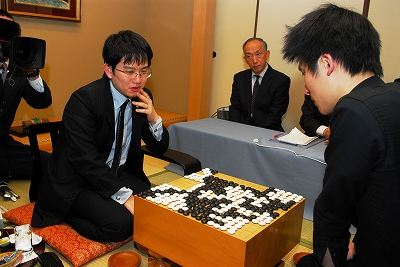Here is the record of the forth game. Yamashita Keigo, playing white, won by 7.5 points.

(;GMFFCA[UTF-8]AP[CGoban:3]ST RU[Japanese]SZKM[6.50] GN[Kisei Title 2010, game 4]PW[Yamashita Keigo]PB[Cho U]WR[9p]BR[9p]DT[2010-02-19]EV[Kisei Title 2010]RE[W+7.50]MULTIGOGM ;B[pd] ;W[dp] ;B[qp] ;W[dd] ;B[fq] ;W[cn] ;B[lq] (;W[pm] ;B[pk] ;W[op] (;B[qn] ;W[mp]LB[qm:A][pn:B]C[Since A and B are miai for black, white decided to change the course and possibly decide between A and B later, depending on black's response on the lower side.] ;B[lp] ;W[lo] ;B[mq] (;W[qq] (;B[rq] ;W[ko] (;B[iq] (;W[pq] ;B[pn] ;W[rr] ;B[om] ;W[nc] ;B[np] ;W[no] ;B[mo] ;W[mn] ;B[oo] ;W[mp] ;B[nq] ;W[rp] ;B[ro] ;W[sq] ;B[lc] ;W[ld] ;B[kd] ;W[md] ;B[jc] ;W[pf] ;B[of] ;W[qc] ;B[qd] ;W[pc] ;B[od] ;W[oc] ;B[pg] ;W[gc] ;B[kf] ;W[ce] ;B[mf] ;W[lk] ;B[dm] ;W[cm] ;B[dk] ;W[gp] ;B[dq] ;W[cq] ;B[cp] ;W[do] ;B[cr] ;W[bq] ;B[er] ;W[br] ;B[dh] ;W[lg] ;B[lf] ;W[je] ;B[he] ;W[hd] ;B[ge] ;W[ke] ;B[ie] ;W[id] ;B[jd] ;W[jf] ;B[fc] ;W[gd] ;B[fd] ;W[fb] ;B[jg] ;W[ig] ;B[fg] ;W[ih] ;B[ec] ;W[hb] ;B[dc] ;W[cc] ;B[cb] ;W[ff] ;B[gf] ;W[jb] ;B[bc] ;W[bd] ;B[cd] ;W[hq] ;B[ip] ;W[ir] ;B[jr] ;W[gr] ;B[is] ;W[hr] ;B[eo] ;W[go] ;B[fo] ;W[hn] ;B[in] ;W[im] ;B[jn] ;W[jm] ;B[kn] ;W[km] ;B[jp] ;W[fm] ;B[fn] ;W[gm] ;B[dn] ;W[co] ;B[cl] ;W[cc] ;B[bm] ;W[df] ;B[fe] ;W[ep] ;B[fp] ;W[eq] ;B[if] ;W[le] ;B[kg] ;W[ch] ;B[ci] ;W[bi] ;B[di] ;W[bg] ;B[bj] ;W[dg] ;B[ai] ;W[cd] ;B[lm] ;W[gh] ;B[fh] ;W[el] ;B[dl] ;W[nj] ;B[nl] ;W[ll] ;B[ji] ;W[ki] ;B[kj] ;W[lj] ;B[li] ;W[mi] ;B[kh] ;W[ij] ;B[oi] ;W[fi] ;B[fj] ;W[mm] ;B[ln] ;W[ml] ;B[hl] ;W[hk] ;B[il] ;W[jl] ;B[kk] ;W[kl] ;B[gk] ;W[gl] ;B[ik] ;W[jk] ;B[hj] ;W[hm] ;B[hk] ;W[fr] ;B[bo] ;W[bp] ;B[bn] ;W[dr] ;B[rc] ;W[rb] ;B[rd] ;W[ii] ;B[gi] ;W[hi] ;B[ei] ;W[fk] ;B[gj] ;W[jj] ;B[mh] ;W[or] ;B[mo] ;W[ej] ;B[fi] ;W[mp] ;B[nr] ;W[ni] ;B[nh] ;W[kp] ;B[kq] ;W[pp] ;B[mo] ;W[oj] ;B[pj] ;W[mp] ;B[ic] ;W[ib] ;B[mo] ;W[pi] ;B[oh] ;W[mp] ;B[oq] ;W[so] ;B[sn] ;W[pr] ;B[mo] ;W[qo] ;B[rn] ;W[mp] ;B[nd] ;W[mc] ;B[mo] ;W[ef] ;B[ab] ;W[ba] ;B[gg] ;W[mp] ;B[bb] ;W[mo] ;B[lr] ;W[eb] ;B[db] ;W[bh] ;B[sb] ;W[ra] ;B[ek] ;W[ah] ;B[aj] ;W[nk] ;B[bf] ;W[ad] ;B[af] ;W[ee] ;B[cf] ;W[cg] ;B[ed] ;W[ol] ;B[pl] ;W[nm] ;B[on] ;W[be] ;B[ns] ;W[ki] ;B[kj] ;W[ao] ;B[eg] ;W[ae] ;B[os] ;W[hs] ;B[js] ;W[me] ;B[ne] ;W[an] ;B[bl] ;W[da] ;B[ca] ;W[ea] ;B[ps] ;W[qs] ;B[am] ;W[ap] ;B[hg] ;W[hh] ;B[ok] ;W[po] ;B[fl] ;W[em] ;B[en] ;W[nl] ;B[fk] ;W[sp]) (;W[qm] ;B[pn] (;W[om] ;B[on] ;W[nm] ;B[nn] ;W[mn] ;B[no]) (;W[on] ;B[om] ;W[ol] ;B[nm] ;W[pl] ;B[nn])) (;W[nc] ;B[pf] ;W[jd] ;B[on] ;W[mo] ;B[om])) (;B[pn] ;W[on] (;B[np] ;W[no] ;B[mo] ;W[mn] ;B[oq] ;W[mp] ;B[nq] ;W[qm]) (;B[om] ;W[jq] ;B[np] ;W[mo] ;B[oq] ;W[no] ;B[nq] ;W[ol] ;B[nm] ;W[pl])) (;B[on] ;W[pn] ;B[po] ;W[oo] ;B[om] ;W[jq] ;B[pq] ;W[oq] ;B[qr] ;W[or] ;B[jr] ;W[ir] ;B[iq] ;W[ip] ;B[hq] ;W[kr] ;B[jp] ;W[kq] ;B[hr] ;W[kp] ;B[dr])) (;B[ko] (;W[pp] ;B[ro] ;W[pn] ;B[ql] ;W[kn] ;B[jo] ;W[jn] ;B[io] ;W[mo]) (;W[qm]))) (;W[mo]) (;W[ko] (;B[np] ;W[no] ;B[mo] ;W[mn] (;B[oq] ;W[mp] ;B[nq] ;W[qm]) (;B[pn] (;W[on] ;B[oq] ;W[mp] ;B[nq]) (;W[nq] ;B[mp] ;W[on]))) (;B[pn] ;W[on] ;B[np] ;W[oq]))) (;B[oo] ;W[no] ;B[po] ;W[nn] ;B[np] ;W[mp] ;B[nq] ;W[mq]LB[lq:A]C[Black's stone A would be hurt badly this way.])) (;W[ip]C[Also possible.]))

Next game will be played on February 25th and 26th. You can find the complete tournament table here.

### Cho U Leads 3-0 in Kisei Title

Friday, February 5th, 2010

Cho U seems to be back in good shape after a bad year: he is just one win away from capturing the Kisei title from Yamashita Keigo.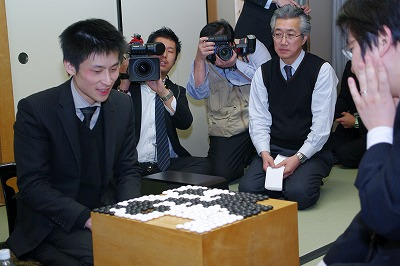Here is the record of the third game. Cho U, white, won by 8.5 points after a very confusing multi-step ko fight involving a large black group.

(;GMFFCA[UTF-8]AP[CGoban:3]ST RU[Japanese]SZKM[6.50] PW[Cho U]PB[Yamashita Keigo]WR[9p]BR[9p]DT[2010-02-03,04]EV[34th Kisei title match 3]RE[W+8.50] ;B[pd] ;W[dp] (;B[qp] (;W[ed] (;B[op] ;W[cd] (;B[pj] (;W[nc] ;B[pf] (;W[kd] ;B[dj] (;W[cl] (;B[jp] (;W[ek] (;B[fi] (;W[hj] ;B[df] ;W[ej] ;B[ei] ;W[di] ;B[dh] ;W[ci] ;B[fe] ;W[fd] ;B[ge] ;W[gd] ;B[he] ;W[hd] ;B[gk] ;W[hk] ;B[gl] ;W[hl] ;B[el] ;W[dk] ;B[gj] ;W[hn] ;B[ch] ;W[bi] ;B[je] ;W[jd] ;B[fp] ;W[hp] ;B[en] ;W[lp] ;B[ko] ;W[np] ;B[nq] ;W[oo] ;B[pp] ;W[mq] ;B[no] ;W[mo] ;B[mp] ;W[gm] ;B[fm] ;W[np] ;B[nn] ;W[nr] ;B[mp] ;W[gi] ;B[np] ;W[fj] ;B[gh] ;W[hi] ;B[cn] ;W[bp] ;B[dq] ;W[cq] ;B[eq] ;W[kq] ;B[co] ;W[cp] ;B[gn] ;W[hm] ;B[dr] ;W[cr] ;B[go] ;W[ho] ;B[bo] ;W[gp] ;B[gq] ;W[fr] ;B[fq] ;W[hq] ;B[gr] ;W[fo] ;B[eo] ;W[fn] ;B[ep] ;W[bm] ;B[ao] ;W[lo] ;B[mr] ;W[lr] ;B[lq] ;W[br] ;B[aq] ;W[mq] ;B[ce] ;W[bd] ;B[lq] ;W[hg] ;B[gg] ;W[hh] ;B[ke] ;W[le] ;B[lf] ;W[mf] ;B[lg] ;W[mg] ;B[ie] ;W[lh] ;B[kh] ;W[me] ;B[oc] ;W[nb] ;B[mq] ;W[qh] ;B[qj] ;W[pg] ;B[nd] ;W[qf] ;B[qe] ;W[rf] ;B[lc] ;W[lb] ;B[md] ;W[kc] ;B[of] ;W[ld] ;B[re] ;W[pb] ;B[qc] ;W[ne] ;B[kp] ;W[qb] ;B[rb] ;W[ra] ;B[oe] ;W[rc] ;B[rd] ;W[sb] ;B[pc] ;W[hr] ;B[jr] ;W[ap] ;B[cs] ;W[bs] ;B[ar] ;W[ds] ;B[gs] ;W[jq] ;B[kr] ;W[hs] ;B[es] ;W[dm] ;B[em] ;W[fl] ;B[jg] ;W[nh] ;B[mk] ;W[cm] ;B[cs] ;W[gf] ;B[eh] ;W[ds] ;B[do] ;W[bn] ;B[cs] ;W[nj] ;B[nk] ;W[ds] ;B[bq] ;W[an] ;B[cs] ;W[oj] ;B[ok] ;W[ds] ;B[fk] ;W[fl] ;B[cs] ;W[fg] ;B[fh] ;W[ds] ;B[fk] ;W[gk] ;B[cs] ;W[rj] ;B[rk] ;W[ds] ;B[dd] ;W[dl] ;B[cs] ;W[ee] ;B[ef] ;W[ds] ;B[go] ;W[gn] ;B[cs] ;W[hf] ;B[ff] ;W[ds] ;B[dc] ;W[dn] ;B[cs] ;W[as] ;B[aq] ;W[er] ;B[bq] ;W[ds] ;B[fs] ;W[er] ;B[cs] ;W[ar] ;B[id] ;W[ic] ;B[hc] ;W[hb] ;B[gc] ;W[fc] ;B[bq] ;W[ds] ;B[gb] ;W[fr] ;B[fb] ;W[be] ;B[bf] ;W[ae] ;B[cc] ;W[bc] ;B[bb] ;W[af] ;B[bg] ;W[ag] ;B[ah] ;W[bh] ;B[ac] ;W[ai] ;B[pi] ;W[ri] ;B[og] ;W[ph] ;B[jl] ;W[sk] ;B[rl] ;W[ib] ;B[iq] ;W[jn] ;B[kn] ;W[jm] ;B[kl] ;W[eb] ;B[ec] ;W[ga] ;B[de] ;W[fa] ;B[db] ;W[ea] ;B[sl] ;W[oh] ;B[li] ;W[km] ;B[lm] ;W[mc] ;B[mh] ;W[sf] ;B[ij] ;W[ji] ;B[jj] ;W[ii] ;B[ob] ;W[oa] ;B[ki] ;W[il] ;B[sj] ;W[od] ;B[nd] ;W[mj] ;B[lj] ;W[jk] ;B[kk] ;W[ik] ;B[si] ;W[sh] ;B[sk] ;W[ng] ;B[da] ;W[ha] ;B[qi] ;W[rh] ;B[kj] ;W[if] ;B[jf] ;W[js] ;B[ks] ;W[ip] ;B[ir] ;W[jo] ;B[oi] ;W[ni] ;B[is] ;W[mi] ;B[lh]) (;W[dh] (;B[di] ;W[eh] (;B[fk] ;W[fl] ;B[gk] ;W[gl] ;B[hk] ;W[hl] ;B[ik] ;W[jm]) (;B[fj] ;W[gl]) (;B[dk] ;W[dl] ;B[el] ;W[fk] ;B[fj] ;W[em] ;B[fl] ;W[gk])) (;B[ej] ;W[fk])) (;W[gk] ;B[hi] ;W[dh] ;B[ej]) (;W[gj] ;B[gi] ;W[hj] ;B[hi] ;W[ij] ;B[jh] ;W[kj] ;B[id])) (;B[dg] ;W[ef] ;B[fi]) (;B[ej] ;W[fk] ;B[fj] ;W[gk])) (;W[fq]) (;W[dh] ;B[ek] ;W[dn])) (;B[dg] ;W[jp]) (;B[ek] ;W[jq])) (;W[jq] ;B[cn] ;W[fp])) (;W[pb]) (;W[jp]) (;W[jc])) (;W[dj]) (;W[cj])) (;B[cn] ;W[ck])) (;B[cd] (;W[cc]) (;W[df])) (;B[cc])) (;W[dc] ;B[fq] ;W[cn] ;B[lq] ;W[de] ;B[pj] ;W[nd] ;B[lc] ;W[me] ;B[qf]) (;W[dd] ;B[op]) (;W[op])) (;B[pq] ;W[dd] ;B[fq] ;W[ip] ;B[dn] ;W[fp] ;B[cq] ;W[eq] ;B[cp] ;W[cl] ;B[bn] ;W[gq]))

Next game will be played on February 18th and 19th.

The complete tournament table is here.

### Kisei Title Started: Cho U Won Game One

Friday, January 15th, 2010

Cho U, challenger in this year’s Kisei title match, won game one against title holder Yamashita Keigo.

In their most recent encounter, Yamashita captured the Tengen title from Cho U last month.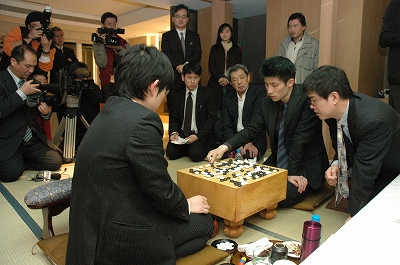The record of game one is here. Cho U, playing white, won by resignation.

(;GMFFCA[UTF-8]AP[CGoban:3]ST RU[Japanese]SZKM[6.50] GN[34th Kisei Title Match, game 1]PW[Cho U]PB[Yamashita Keigo]WR[9p]BR[9p]DT[2010-01-14,15]EV[34th Kisei Title Match]RE[W+Resign]MULTIGOGM ;B[qd] ;W[pp] ;B[dd] (;W[dp] (;B[qn] (;W[pk] (;B[np] ;W[pn] (;B[qq] (;W[qo] ;B[pq] (;W[lq] (;B[nr] (;W[qm] (;B[fq] (;W[cn] (;B[dr] (;W[gq] (;B[gp] ;W[hq] (;B[hp] (;W[fr] (;B[eq] (;W[ip] (;B[fo] (;W[ck] (;B[oc] (;W[mq] (;B[iq] ;W[ir] ;B[jq] ;W[jp] ;B[nq] ;W[jr] ;B[cq] ;W[rp] ;B[rq] ;W[cp] ;B[er] ;W[fc] ;B[hc] ;W[df] ;B[ec] ;W[fd] ;B[ee] ;W[eb] ;B[db] ;W[ed] ;B[de] ;W[dc] ;B[cc] ;W[ge] ;B[jd] ;W[qf] ;B[oe] ;W[cf] ;B[ef] ;W[dh] ;B[eh] ;W[di] ;B[ec] ;W[gg] ;B[pg] ;W[rd] ;B[rc] ;W[dc] ;B[ei] ;W[gi] ;B[ej] ;W[pd] ;B[qc] ;W[qh] ;B[dk] ;W[cl] ;B[ig] ;W[hg] ;B[eg] ;W[if] ;B[jf] ;W[jg] ;B[ih] ;W[je] ;B[ie] ;W[kf] ;B[hf] ;W[jf] ;B[he] ;W[kd] ;B[gf] ;W[jc] ;B[id] ;W[hb] ;B[ic] ;W[ib] ;B[kc] ;W[jb] ;B[ld] ;W[ke] ;B[lc] ;W[gc] ;B[fg] ;W[jh] ;B[ii] ;W[ji] ;B[jj] ;W[kj] ;B[ij] ;W[kk] ;B[li] ;W[ki] ;B[qg] ;W[rg] ;B[rh] ;W[rf] ;B[ec] ;W[ga] ;B[ri] ;W[pf] ;B[pi] ;W[qj] ;B[qi] ;W[og] ;B[ph] ;W[of] ;B[ni] ;W[od] ;B[nd] ;W[ne] ;B[pc] ;W[oh] ;B[ok] ;W[oi] ;B[pj] ;W[oj] (;B[qk] ;W[pl] ;B[rl] ;W[md] ;B[nc] ;W[il] ;B[me] ;W[nf] ;B[nn] ;W[nm] ;B[jm] ;W[hk] ;B[cj] ;W[bi] ;B[jl] ;W[jk] ;B[ik] ;W[im] ;B[in] ;W[jn] ;B[kn] ;W[jo] ;B[mm] ;W[nl] ;B[ll] ;W[hh] ;B[fk] ;W[gl] ;B[bj] ;W[dj] ;B[ci] ;W[ch] ;B[bh] ;W[dl] ;B[ek] ;W[bk] ;B[ai] ;W[mo] ;B[no] ;W[mn] ;B[mp] ;W[lo] ;B[bq] ;W[bp] ;B[ap] ;W[ao] ;B[aq] ;W[bg] ;B[dg] ;W[cg] ;B[ce] ;W[af] ;B[ah] ;W[al] ;B[bo] ;W[aj] ;B[ak] ;W[sq] ;B[sr] ;W[aj] ;B[om] ;W[ol] ;B[ak] ;W[rk] ;B[rj] ;W[aj] ;B[ea] ;W[fb] ;B[ak] ;W[sp] ;B[lp] ;W[ko] ;B[rr] ;W[aj] ;B[hm] ;W[hl] ;B[ak] ;W[an] ;B[bn] ;W[aj] ;B[kq] ;W[kp] ;B[ak] ;W[am] ;B[bm] ;W[aj] ;B[kr] ;W[lr] ;B[ak] ;W[bl] ;B[em] ;W[aj] ;B[ks] ;W[ls] ;B[ak] ;W[dn] ;B[eo] ;W[aj] ;B[da] ;W[bi]C[Black resigned.]) (;B[pl] ;W[qk] ;B[ql] ;W[ol] ;B[rk] ;W[nk] ;B[rj])) (;B[nq] ;W[cq]) (;B[cq])) (;W[cq] ;B[iq] ;W[ir] ;B[jq] ;W[jr] ;B[jp] ;W[io] ;B[jo] ;W[in] ;B[jn] ;W[im] ;B[kr])) (;B[iq] ;W[ir] ;B[jq] ;W[jr] ;B[jp] ;W[mq] (;B[io] ;W[nq] ;B[oq] ;W[oc]) (;B[nq] ;W[io] ;B[jo] ;W[in] ;B[lo] ;W[ls])) (;B[jp] (;W[io] ;B[iq] ;W[ir] ;B[jq] ;W[jr] ;B[jo] ;W[in] ;B[jn] ;W[im] ;B[kr]) (;W[iq] ;B[io] ;W[jo] ;B[kp] ;W[kr] ;B[jn] ;W[ko] ;B[lp]))) (;W[io] ;B[cq] ;W[ck]) (;W[mq] ;B[nq] ;W[cq] ;B[iq] ;W[ir] ;B[jq] ;W[jr] ;B[kq] ;W[kr] ;B[io] (;W[jp] ;B[kp] ;W[jo] ;B[lp] ;W[in] ;B[mp] ;W[ho] ;B[fm] ;W[dk] ;B[lr] ;W[er] ;B[dq] ;W[gs] ;B[hm] ;W[jl]) (;W[ck]))) (;B[cl] ;W[dl] ;B[dk] ;W[dm] (;B[fo] ;W[ck] ;B[cj] ;W[bk] ;B[dj] ;W[bl] ;B[kc]) (;B[cq] ;W[fp] ;B[ek] ;W[er] ;B[dq] ;W[fo])) (;B[iq] ;W[ir] ;B[jq] ;W[jr] ;B[kq] (;W[kr] ;B[io] ;W[jp] ;B[kp] ;W[jo] ;B[lp] ;W[fp]) (;W[io] ;B[kr] ;W[fp] ;B[hn] ;W[ep])) (;B[io] ;W[jp] ;B[fo] ;W[ck])) (;W[iq] ;B[cq] ;W[ck])) (;B[iq] ;W[ir] ;B[jr] ;W[ip] ;B[jq] ;W[eq] ;B[fp] ;W[er])) (;W[iq])) (;B[fp] ;W[cq]) (;B[cq] ;W[fr] ;B[eq] ;W[hp] ;B[fo] ;W[ck]) (;B[fr] ;W[cq] ;B[hp] ;W[iq] ;B[ep] (;W[jo]) (;W[oc] ;B[jo])) (;B[ep] ;W[dq] ;B[cr] (;W[bq] ;B[eq] ;W[hp] ;B[gn] ;W[ck]) (;W[eo] ;B[eq] ;W[fo] ;B[cp] ;W[do] ;B[bo]))) (;B[gr] ;W[fr] (;B[gp] ;W[hq] ;B[hr] ;W[eq] ;B[fp] ;W[er] ;B[iq]) (;B[er] ;W[fp] ;B[fs] ;W[hq]))) (;W[cq] ;B[iq]) (;W[hq] ;B[cq] ;W[eq] ;B[er] ;W[fp] ;B[gp] ;W[gq] ;B[fr] ;W[go] ;B[ci])) (;B[iq]LB[dr:A])) (;W[hp]) (;W[eq] ;B[fp] ;W[dn] ;B[ip] ;W[ko])) (;B[oc] (;W[fq] ;B[cf]) (;W[cf] ;B[fc] ;W[bd])) (;B[lc])) (;W[rp]) (;W[od] (;B[oc] ;W[pd] ;B[pc] ;W[qe] ;B[nc] ;W[rd] ;B[qc] ;W[pf] ;B[qm] ;W[pm] ;B[ro] ;W[ql] ;B[qp]) (;B[pf] ;W[qc] ;B[rc] ;W[pc] ;B[re] ;W[lc] ;B[qm] ;W[pm] ;B[ql]))) (;B[mq] ;W[lp] ;B[oc] ;W[no]) (;B[qm] (;W[pm] ;B[ro] ;W[ql] ;B[qp]C[Too much to expect for black.]) (;W[rp] ;B[pm] ;W[on] ;B[om] ;W[nn] ;B[nm] ;W[mn] ;B[mq] ;W[lp] ;B[rq] ;W[nk]))) (;W[od] ;B[oc] ;W[pd] ;B[pc] ;W[qe] ;B[nc] (;W[rd] ;B[qc] ;W[pf]) (;W[nd] ;B[md] ;W[qc] ;B[rd] ;W[qb] ;B[rc])) (;W[qm] ;B[oc] (;W[nq] ;B[oq] ;W[mq] ;B[op] ;W[mp]) (;W[oq] ;B[nq]) (;W[lq] ;B[mq] ;W[lp]))) (;W[pq] ;B[pm] ;W[po] ;B[pl] (;W[ok] ;B[nm] ;W[rp]) (;W[qk] (;B[ro] ;W[rq] ;B[nm] ;W[qg]) (;B[ph])))) (;B[pm] ;W[on] ;B[qo] ;W[op] ;B[rq] ;W[om])) (;B[on] ;W[np] ;B[pi]) (;B[qq] ;W[qp] ;B[pq] ;W[op] ;B[rp] ;W[ro] ;B[rq] ;W[qo] ;B[nq]) (;B[pi] ;W[np] ;B[kd]) (;B[fq] ;W[cn] ;B[np] ;W[pn] ;B[pm] ;W[on] ;B[qp] ;W[qo] ;B[ro] ;W[po] ;B[rm] ;W[qq] ;B[rp] ;W[om] ;B[jq])) (;W[nq] (;B[pj]) (;B[kd] (;W[qo] ;B[pn] ;W[pj] ;B[ph] ;W[nj] ;B[mn]) (;W[pf] (;B[od] ;W[qe] ;B[rd] ;W[qj]) (;B[ph] ;W[pd] ;B[pe] ;W[oe] ;B[qe] ;W[qc] ;B[of] ;W[pc] ;B[qf] ;W[nf] ;B[pg] ;W[nd]))) (;B[od])) (;W[np])) (;B[kc])) (;W[cp] ;B[ep] ;W[gq] ;B[cq] ;W[cm] ;B[dn] ;W[bo] ;B[fq] ;W[gp] ;B[bq] ;W[cj] ;B[oc] ;W[qn] ;B[pj] ;W[fc] ;B[hc] ;W[df] ;B[fd] ;W[cc] ;B[cd] ;W[dc] ;B[ed] ;W[fb] ;B[gc] ;W[bc] ;B[nf] ;W[gd]))

The tournament table is here. Game two will be played on January 27 and 28.

### Yamashita Keigo Captured Tengen Title from Cho U

Tuesday, December 22nd, 2009

Yamashita Keigo won the fifth game in the best-of-five Tengen title match and captured the title from Cho U with a 3:2 score.This title match must have been very tensed especially since these two will meet again very soon in an even more important battle: the first game of the Kisei title starts on January 14th with Cho as challenger, and Yamashita as the Kisei title holder.

Cho U had mixed results this year: he lost the Meijin and Tengen titles, but defended Gosei and Oza. Will he be able to capture Kisei for the first time, especially from Yamashita who has been Kisei for four consecutive years?

The 5th and decisive Tengen title game record is here. Yamashita Keigo (black) won by resignation.

(;GMFFCA[UTF-8]AP[CGoban:3]ST RU[Japanese]SZKM[6.50] GN[35th Tengen Title, game 5]PW[Cho U]PB[Yamashita Keigo]WR[9p]BR[9p]DT[2009-12-21]EV[35th Tengen Title, game 5]RO[Title match]RE[B+Resign]MULTIGOGM ;B[pd] ;W[dd] ;B[qp] ;W[op] ;B[lp] ;W[qo] ;B[ro] ;W[po] ;B[rn] ;W[qq] ;B[rq] ;W[pp] ;B[rp] ;W[pl] ;B[qr] ;W[dq] ;B[do] ;W[cm] ;B[cq] ;W[gq] ;B[dp] ;W[dr] (;B[iq] ;W[bo] ;B[bp] ;W[co] ;B[cp] ;W[go] ;B[dm] ;W[cl] (;B[gm] ;W[io] ;B[kq] (;W[hm] (;B[hl] (;W[fm] (;B[fp] ;W[hq] ;B[fl] (;W[gn] ;B[gl] (;W[ln] (;B[im] ;W[ir] (;B[no] (;W[on] (;B[mm] ;W[mn] ;B[nn] ;W[nm] ;B[nl] ;W[om] ;B[np] ;W[nq] ;B[mq] ;W[ml] ;B[lm] ;W[km] ;B[ll] ;W[mk] ;B[kl] ;W[oj] ;B[cj] ;W[dj] ;B[ci] ;W[ck] ;B[em] ;W[di] ;B[cg] ;W[ch] ;B[bh] ;W[dh] ;B[dg] ;W[bi] ;B[bj] ;W[ai] ;B[jr] ;W[ip] ;B[jq] ;W[cr] ;B[er] ;W[eq] ;B[fq] ;W[fr] ;B[gr] ;W[es] ;B[hr] ;W[ep] ;B[fo] ;W[eo] ;B[fn] ;W[dn] ;B[bf] ;W[bk] ;B[cc] ;W[dc] ;B[cd] ;W[hc] ;B[lj] ;W[mj] ;B[jc] ;W[nc] ;B[oc] ;W[nd] ;B[pf] ;W[ie] ;B[ke] ;W[kj] ;B[li] ;W[lk] ;B[kk] ;W[ng] ;B[kh] ;W[kd] ;B[ld] ;W[jd] ;B[lc] ;W[le] ;B[me] ;W[lf] ;B[mf] ;W[mg] ;B[lg] ;W[kf] ;B[jf] ;W[je] ;B[kg] ;W[ke] ;B[oe] ;W[md] ;B[ne] ;W[kc] ;B[lb] ;W[mb] ;B[mi] ;W[ni] ;B[pi] ;W[jj] ;B[ik] ;W[pj] ;B[oh] ;W[ki] ;B[ig] ;W[lh] ;B[mh] ;W[nh] ;B[lh] ;W[ce] ;B[be] ;W[qh] ;B[qi] ;W[og] ;B[pg] ;W[ph] ;B[rh] ;W[qg] ;B[rg] ;W[qf] ;B[qe] ;W[ij] ;B[gg] ;W[gi] ;B[gh] ;W[jk] ;B[jl] ;W[hk] ;B[fi] ;W[il] ;B[jm] ;W[hn] ;B[ik] ;W[ih] ;B[hg] ;W[il] ;B[kn] ;W[fj] ;B[gj] ;W[gk] ;B[ej] ;W[fk] ;B[ok] ;W[ol] ;B[nk] ;W[nj] ;B[qm]) (;B[nn] ;W[np]) (;B[np] ;W[mm] ;B[oq])) (;W[nn] ;B[on] ;W[nm] ;B[oo] ;W[mo] ;B[np] ;W[mp] ;B[mq] ;W[nq] ;B[oq] ;W[nr] ;B[pn] ;W[mr] ;B[lq] ;W[js] ;B[jp])) (;B[cj] ;W[dj] ;B[ci] ;W[ck] (;B[em] ;W[di] ;B[cg] ;W[dh] ;B[bf] ;W[ce] ;B[dg] ;W[eg] ;B[ef] ;W[fg]) (;B[di] ;W[em] ;B[dl] ;W[el] ;B[dk] ;W[dn])) (;B[ip] ;W[in] ;B[jr])) (;B[dl]) (;B[dj]) (;B[cj])) (;W[em] ;B[dl] (;W[dn] ;B[en] ;W[cn] ;B[fn]LB[eo:A]SQ[fp]C[The square marked black stone protects against white's cut at A.]) (;W[el] ;B[dk] ;W[ek] ;B[ej] ;W[fk] ;B[dn] ;W[ln]))) (;W[em] ;B[dl] ;W[el] ;B[dk] ;W[fk] ;B[ck] ;W[gl])) (;B[fl] ;W[gn] ;B[gl] ;W[em] (;B[fp]) (;B[dl] ;W[dn] ;B[en] ;W[cn] ;B[fn] ;W[eo]))) (;W[im] ;B[gn] ;W[ho] ;B[lm]) (;W[gl] ;B[fm] (;W[hk] ;B[il] ;W[im] ;B[dl]) (;W[en] ;B[dn] ;W[em]))) (;B[gl] ;W[ln])) (;W[hq]) (;W[jr]) (;W[ek] ;B[im])) (;B[fm]) (;B[dl])) (;B[go]))

The complete tournament table is here.

### Yamashita Keigo – Cho U 2:1 in Tengen

Wednesday, December 2nd, 2009

Yamashita Keigo won the third game from the Tengen title match, and leads title-holder Cho U 2:1.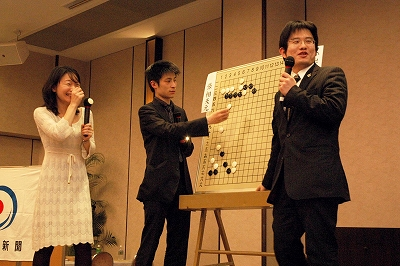The game record is here. Yamashita Keigo (black) won by resignation.

(;CA[Windows-1252]SZAP[MultiGo:4.4.4]GN[Tengen Title 2009]EV[Tengen Title 2009] DT[2009-12-02]PB[Yamashita Keigo]PW[Cho U]KM[6.5]HARE[B+R]MULTIGOGM ;B[qd];W[pp];B[dd];W[cp];B[ep];W[gq];B[cq];W[cm];B[dn];W[bo];B[fq];W[gp];B[bq];W[cj] ;B[oc];W[qn];B[pj];W[fc];B[hc];W[df];B[fd];W[cc];B[cd];W[dc];B[ed];W[fb];B[gc];W[bc] ;B[nf];W[gd];B[id];W[ge];B[ef];W[gg];B[eh];W[gi];B[ej];W[di];B[fh];W[gh];B[fj];W[hk] ;B[dm];W[cl];B[dk];W[je];B[ie];W[jg];B[if];W[kh];B[ff];W[nh];B[ph];W[og];B[nj];W[pg] ;B[qg];W[qf];B[qh];W[pe];B[nd];W[re];B[rd];W[me];B[ne];W[mi];B[cn];W[dl];B[bn];W[el] ;B[gf];W[qk];B[mj];W[kk];B[kj];W[pd];B[pc];W[rc];B[qc];W[lj];B[pk];W[lk];B[ql];W[oq] ;B[kq];W[iq];B[mq];W[mo];B[lo];W[ln];B[ko];W[mp];B[lp];W[rl];B[qm];W[rm];B[pn];W[qj] ;B[qo];W[rn];B[po];W[pm];B[pl];W[om];B[nn];W[nm];B[mn];W[mm];B[on];W[ri];B[lm];W[ml] ;B[ro];W[rq];B[rh];W[oi];B[pi];W[sn];B[si];W[sj];B[qi];W[sh];B[sg];W[np];B[jm];W[sf] ;B[si];W[rj];B[rp];W[sh];B[qq])

Next game will be played on December 10th. You can find the complete tournament table here.